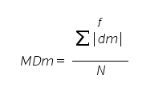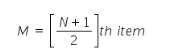# Coefficient of mean deviation from median formula

coefficient of mean deviation from median formula

In order to find out coeffecient of mean deviation from median the following formula is used:
Coefficient of MD from Median = MDm/M
Where:
MDm= Mean Deviation
M= Median